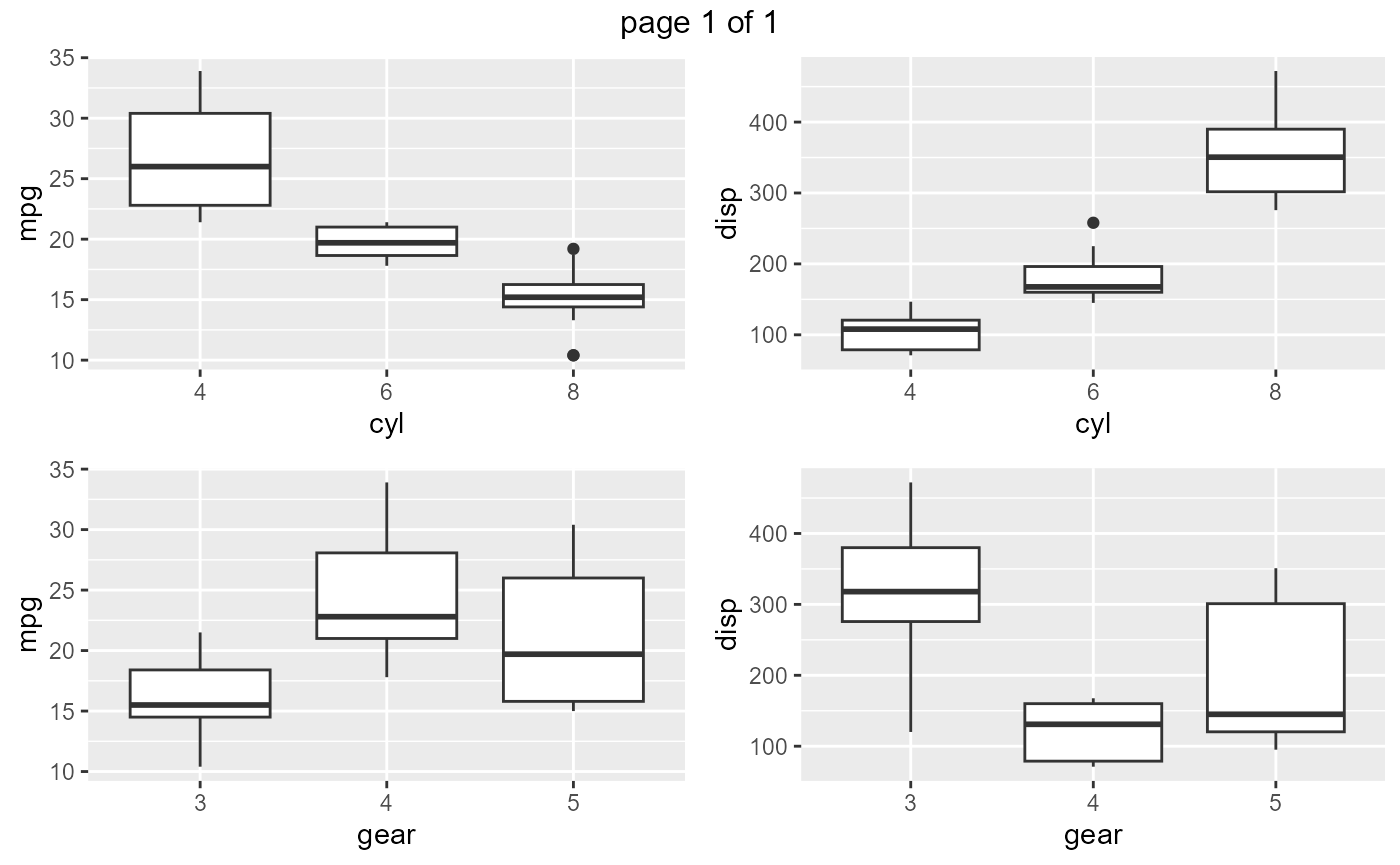Introduction

In this document, we will introduce you to functions for generating different types of plots.

Data

We have modified the mtcars data to create a new data set mtcarz. The only difference between the two data sets is related to the variable types.

str(mtcarz)
#> 'data.frame':    32 obs. of  11 variables:
#>  $mpg : num 21 21 22.8 21.4 18.7 18.1 14.3 24.4 22.8 19.2 ... #>$ cyl : Factor w/ 3 levels "4","6","8": 2 2 1 2 3 2 3 1 1 2 ...
#>  $disp: num 160 160 108 258 360 ... #>$ hp  : num  110 110 93 110 175 105 245 62 95 123 ...
#>  $drat: num 3.9 3.9 3.85 3.08 3.15 2.76 3.21 3.69 3.92 3.92 ... #>$ wt  : num  2.62 2.88 2.32 3.21 3.44 ...
#>  $qsec: num 16.5 17 18.6 19.4 17 ... #>$ vs  : Factor w/ 2 levels "0","1": 1 1 2 2 1 2 1 2 2 2 ...
#>  $am : Factor w/ 2 levels "0","1": 2 2 2 1 1 1 1 1 1 1 ... #>$ gear: Factor w/ 3 levels "3","4","5": 2 2 2 1 1 1 1 2 2 2 ...
#>  \$ carb: Factor w/ 6 levels "1","2","3","4",..: 4 4 1 1 2 1 4 2 2 4 ...

Continuous Data

The following functions will create plots for all or subset of continuous variables in the data set.

Histograms

ds_plot_histogram(mtcarz)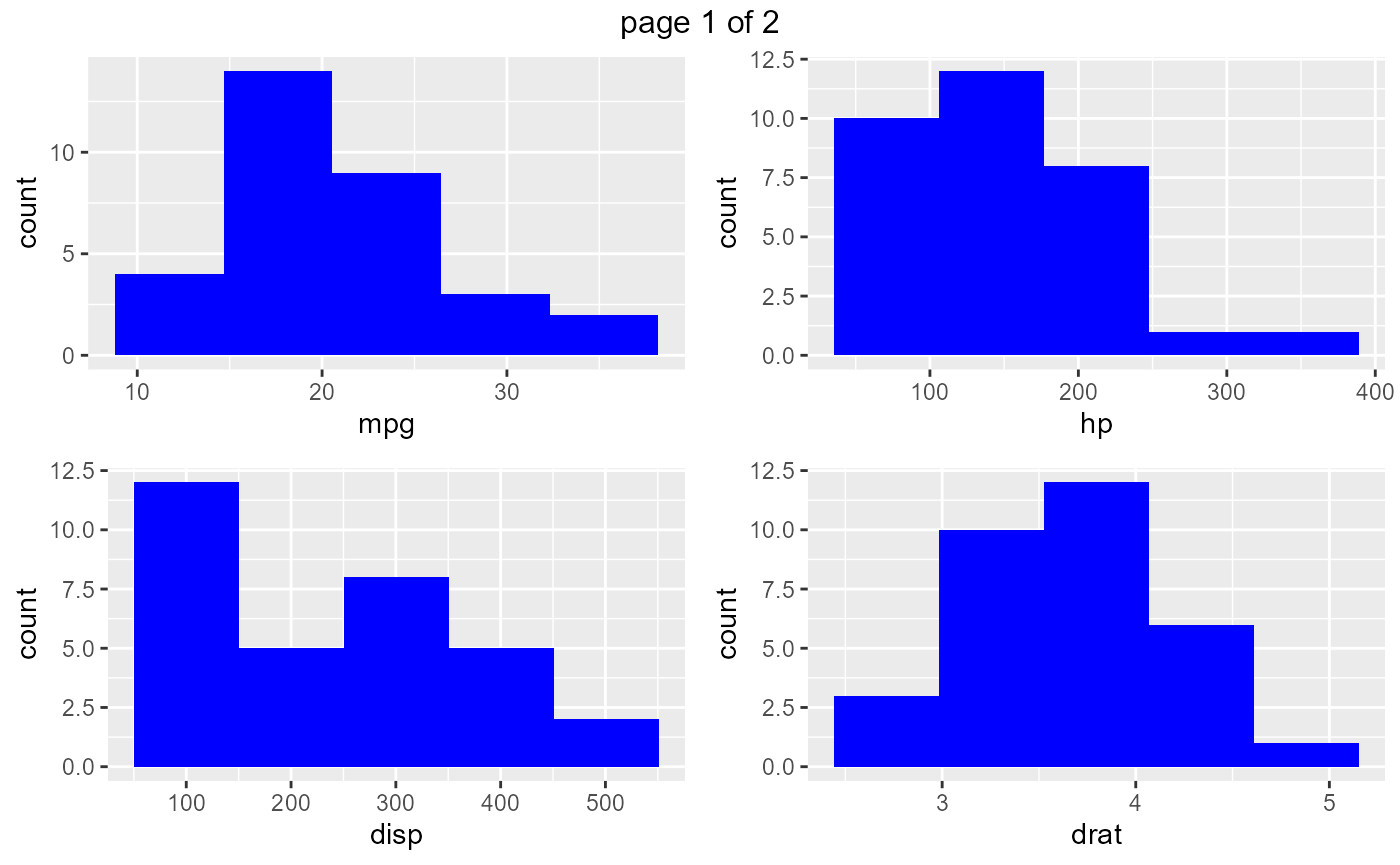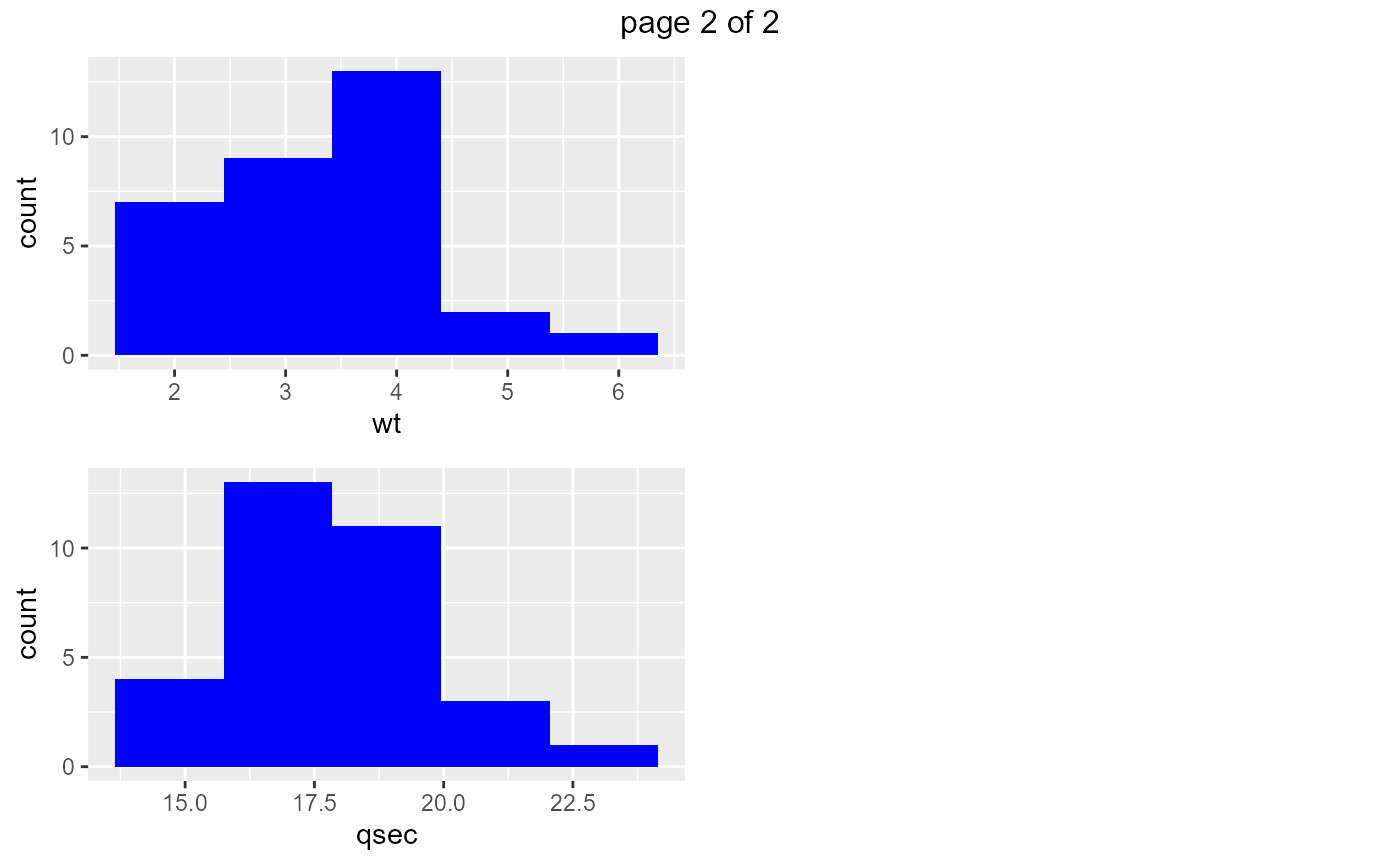Density Plots

ds_plot_density(mtcarz)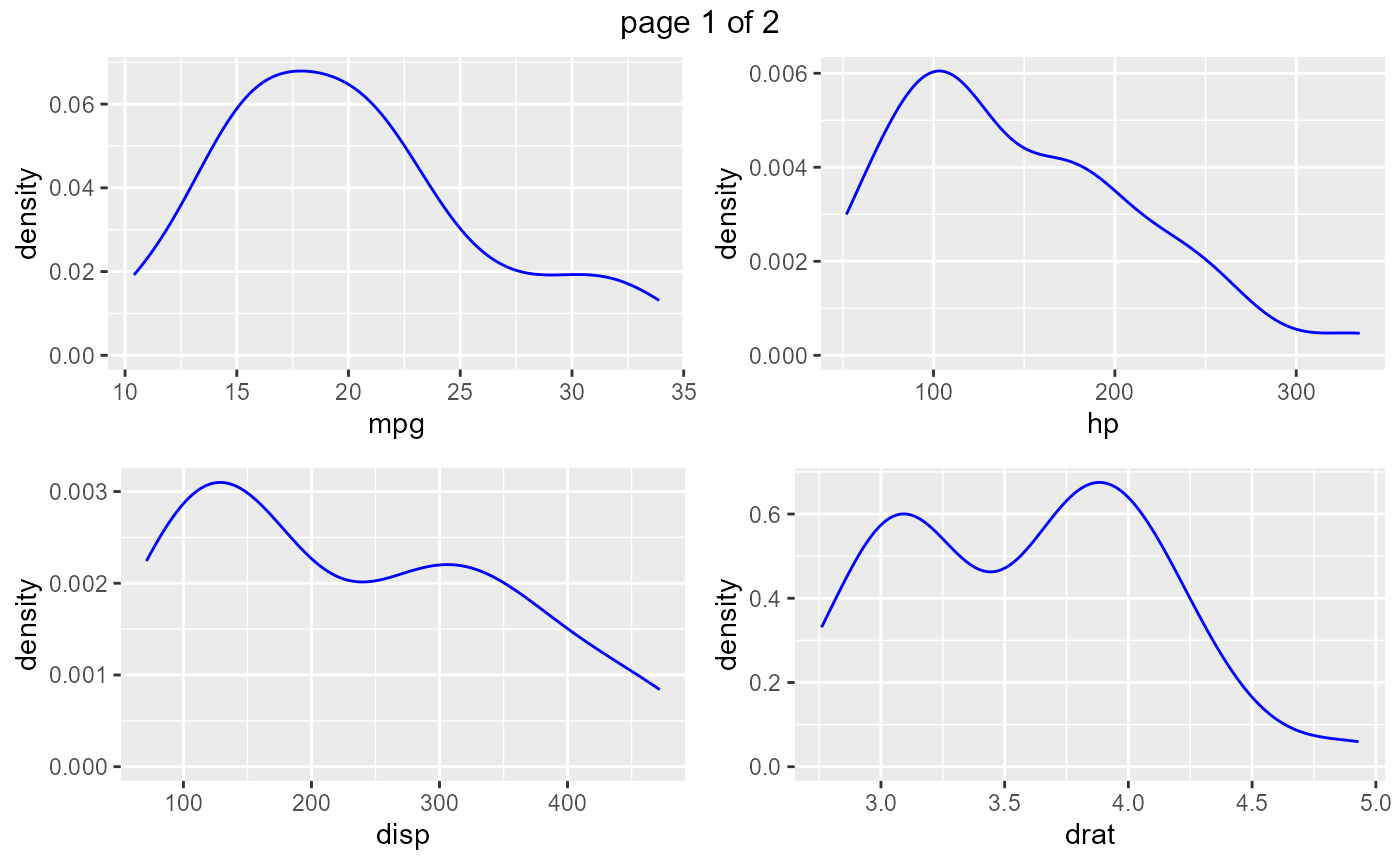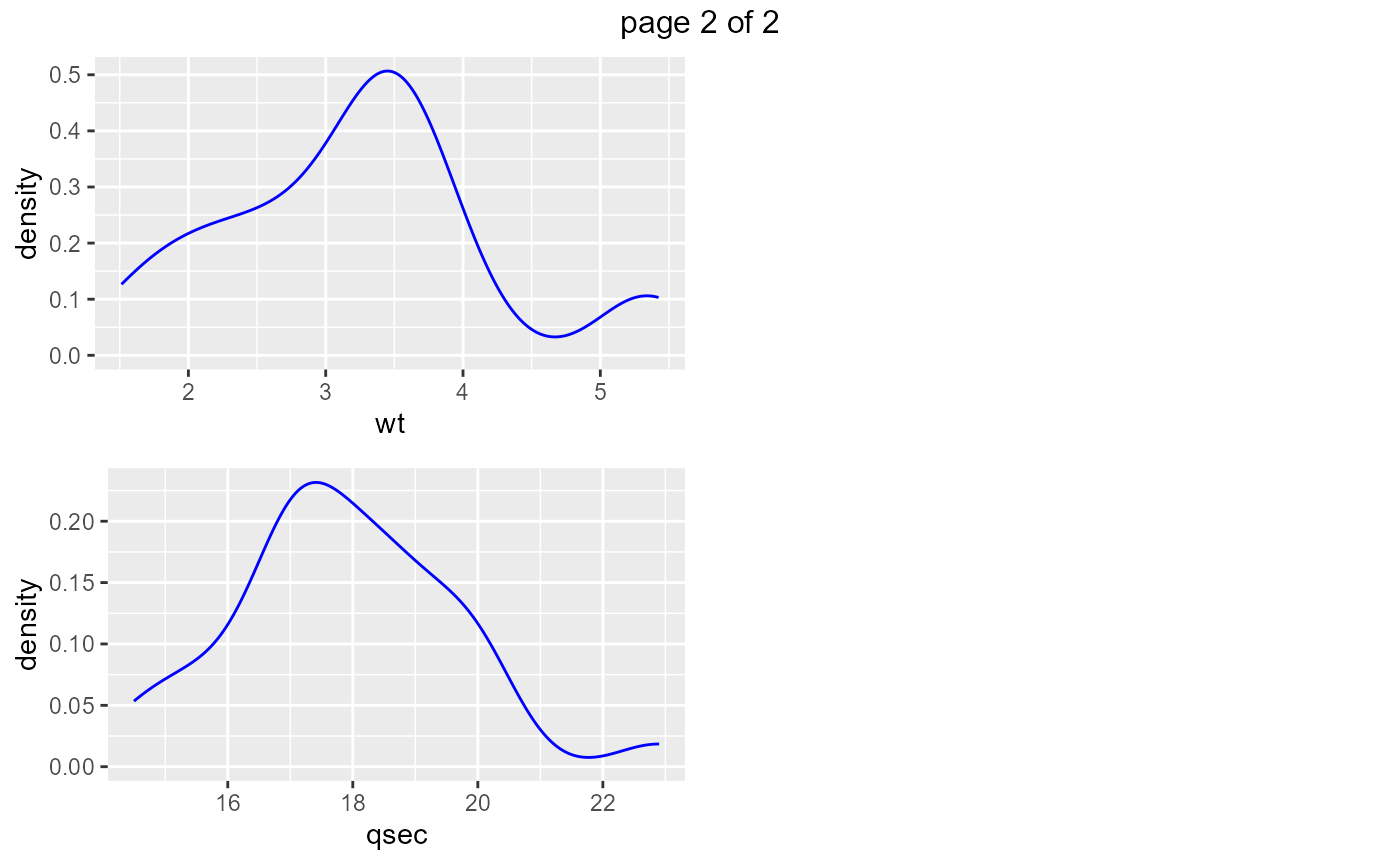Box Plots

ds_plot_box_single(mtcarz)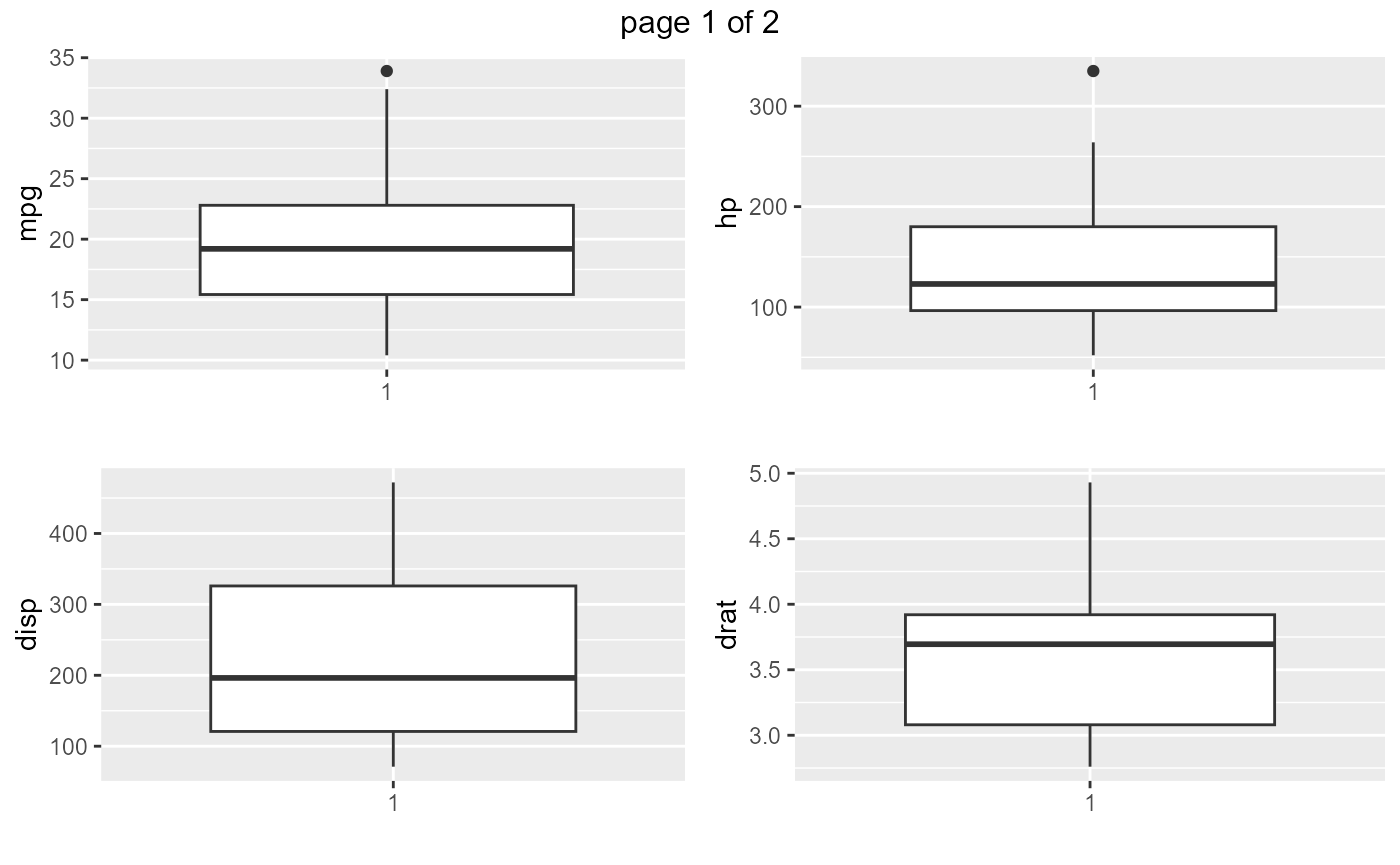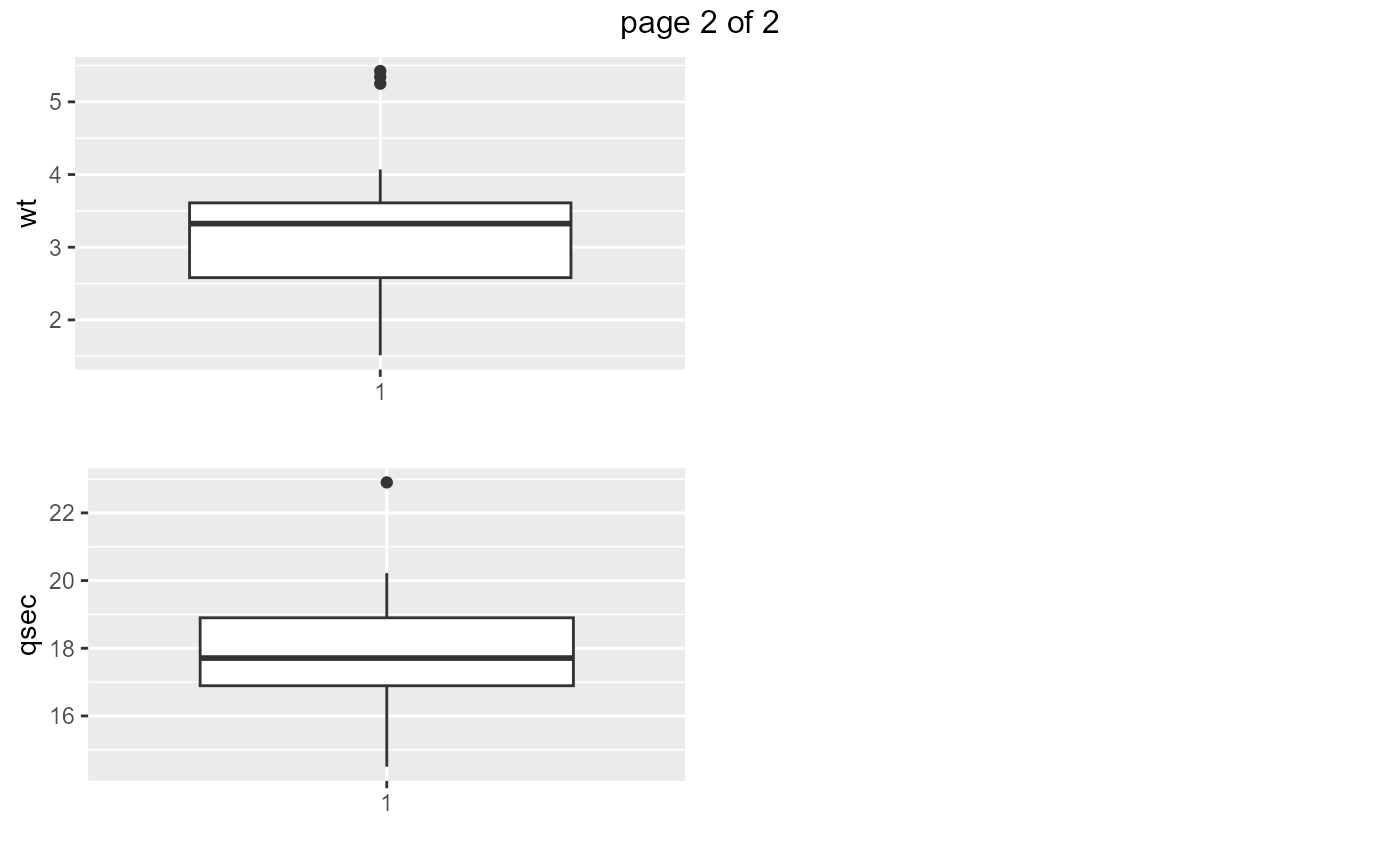Scatter Plots

ds_plot_scatter(mtcarz, mpg, disp, hp)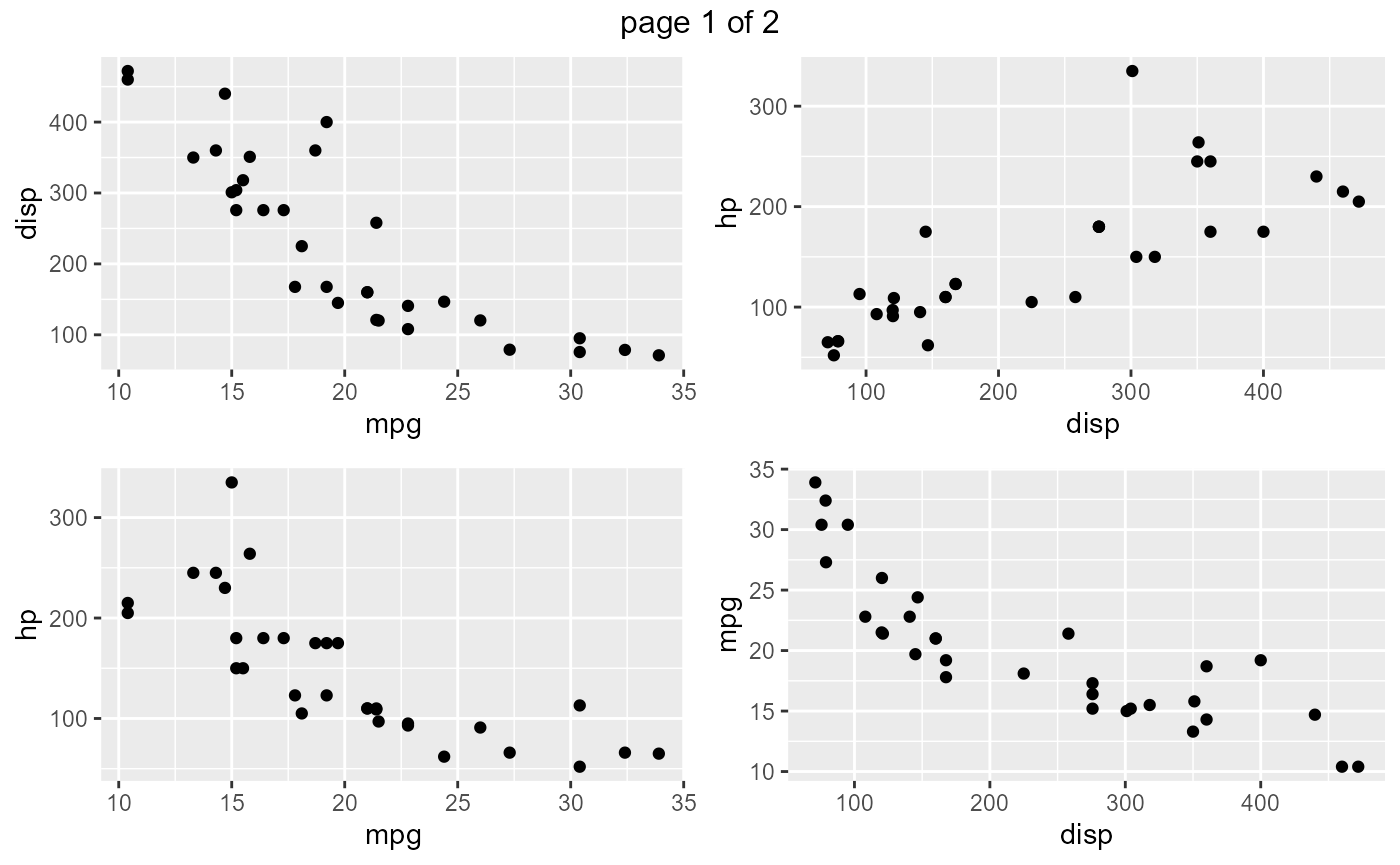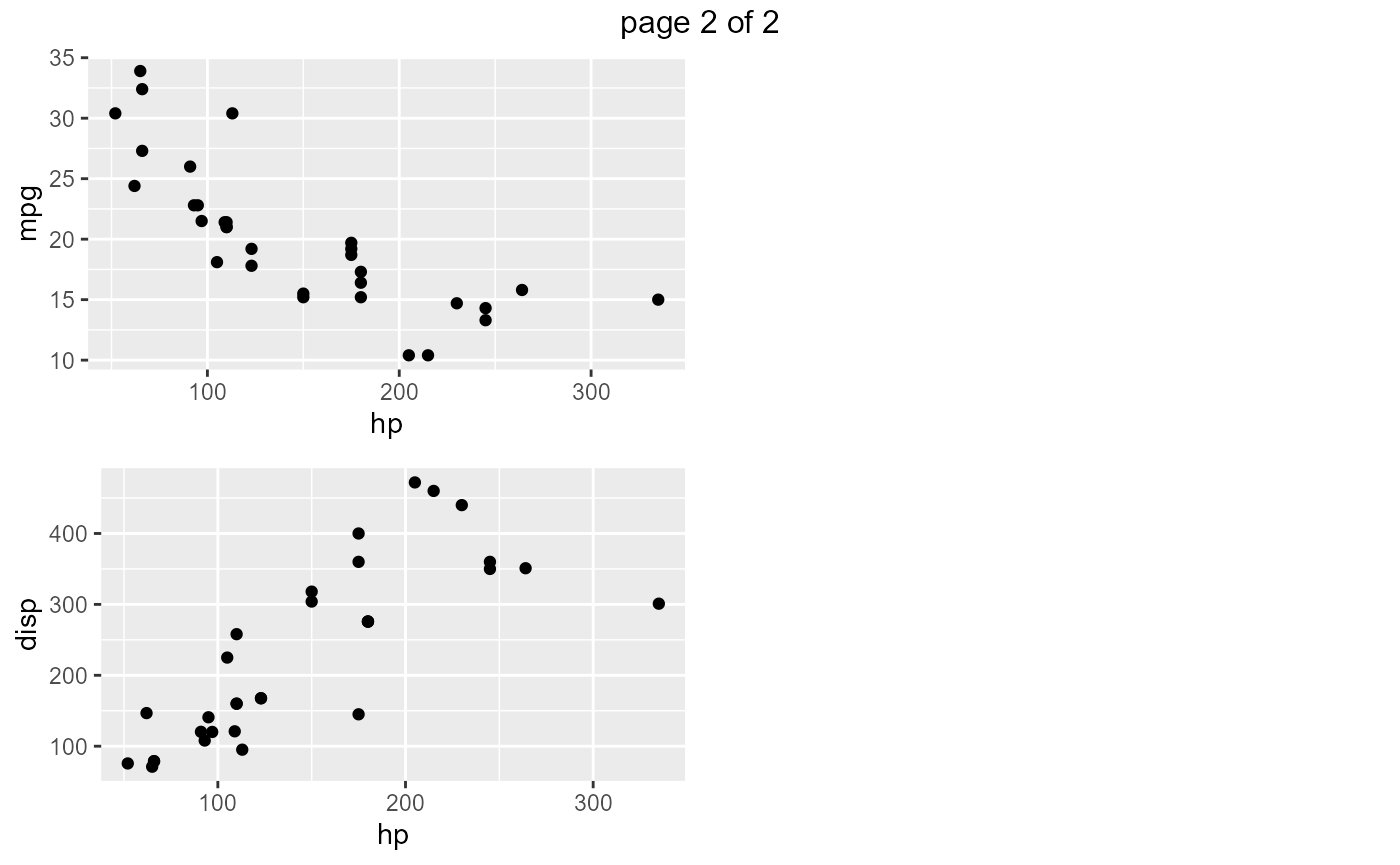Categorical Data

The following functions will create plots for all or subset of categorical variables in the data set.

Bar Plot

ds_plot_bar(mtcarz)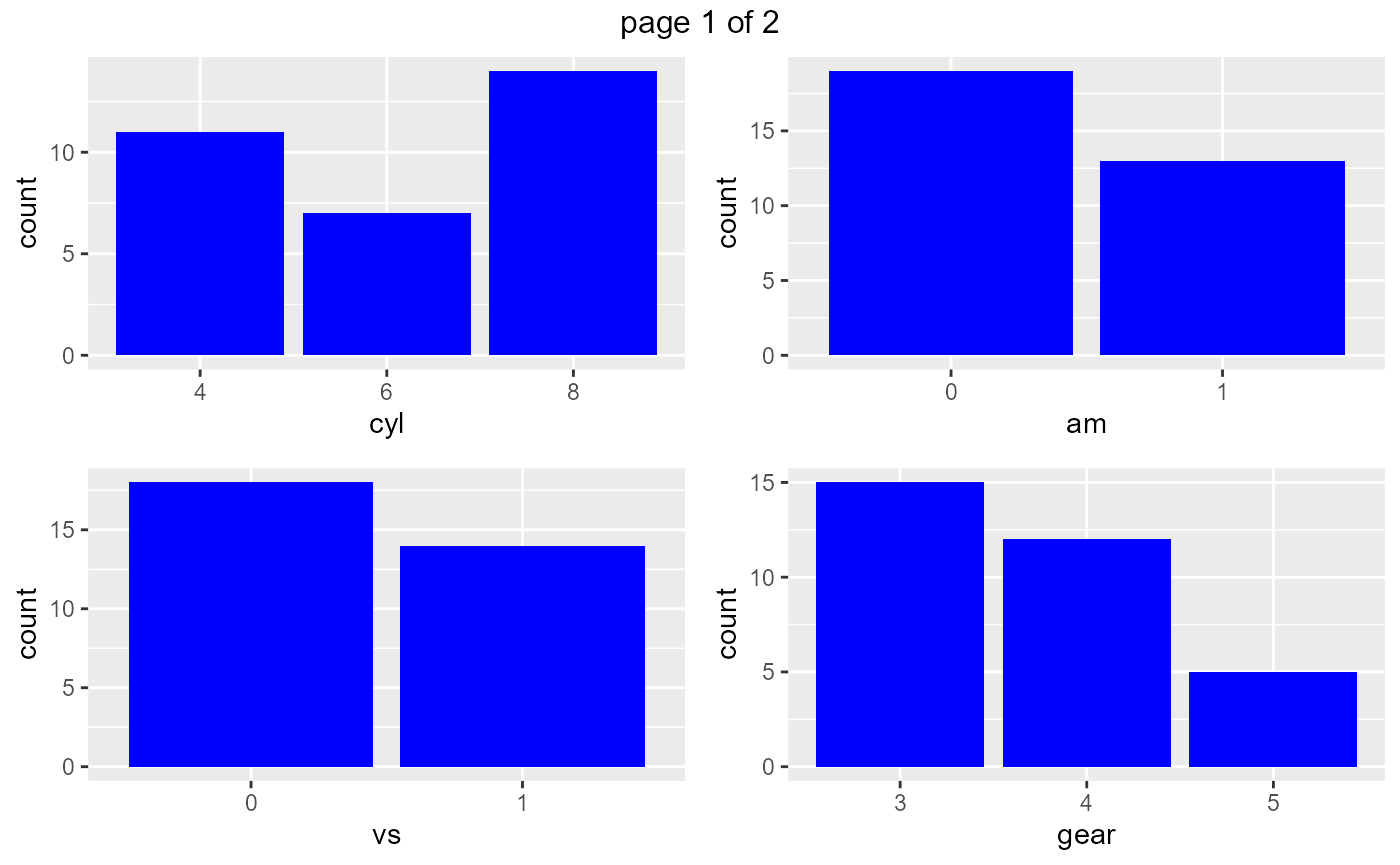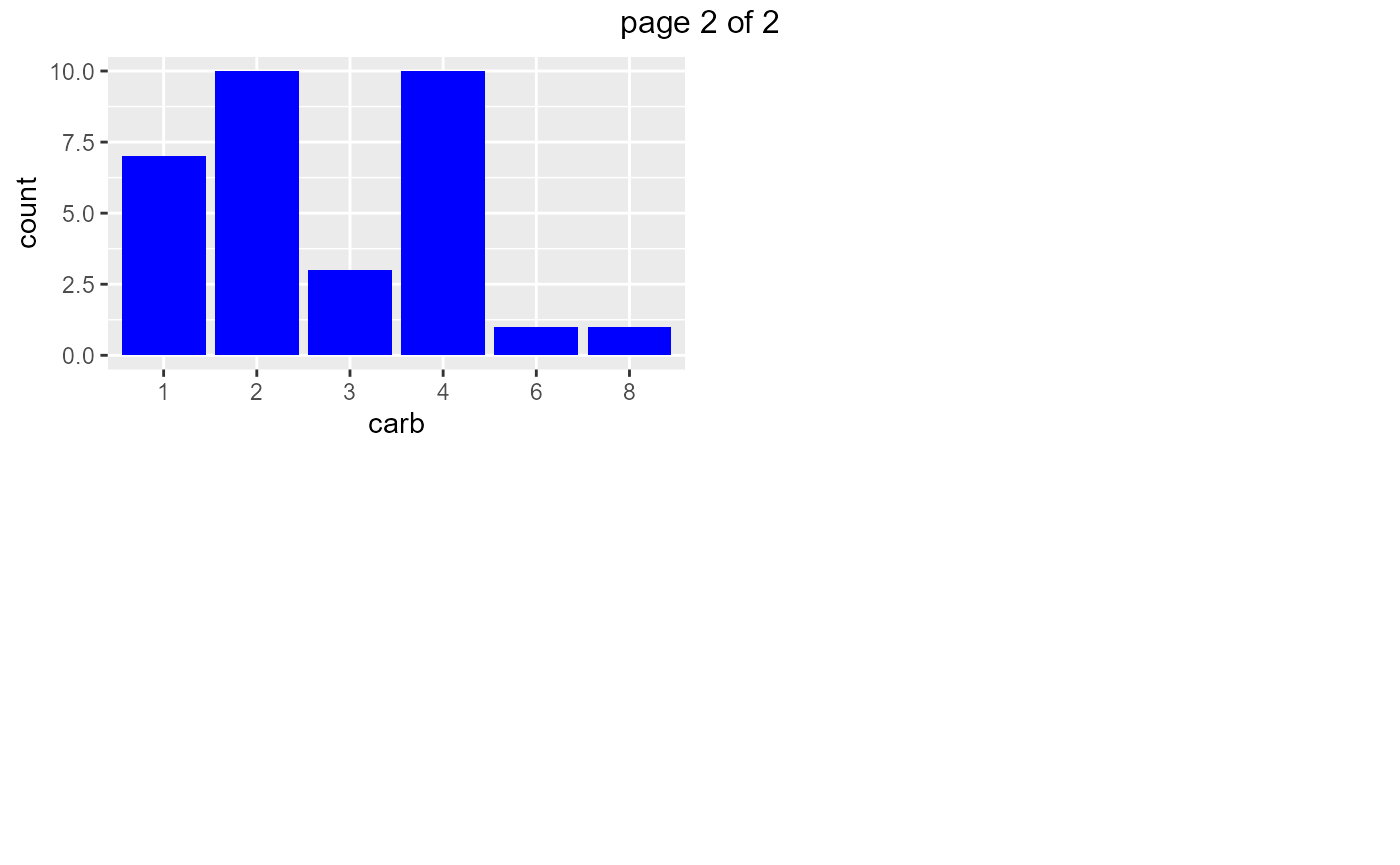Stacked Bar Plot

ds_plot_bar_stacked(mtcarz, cyl, gear, am)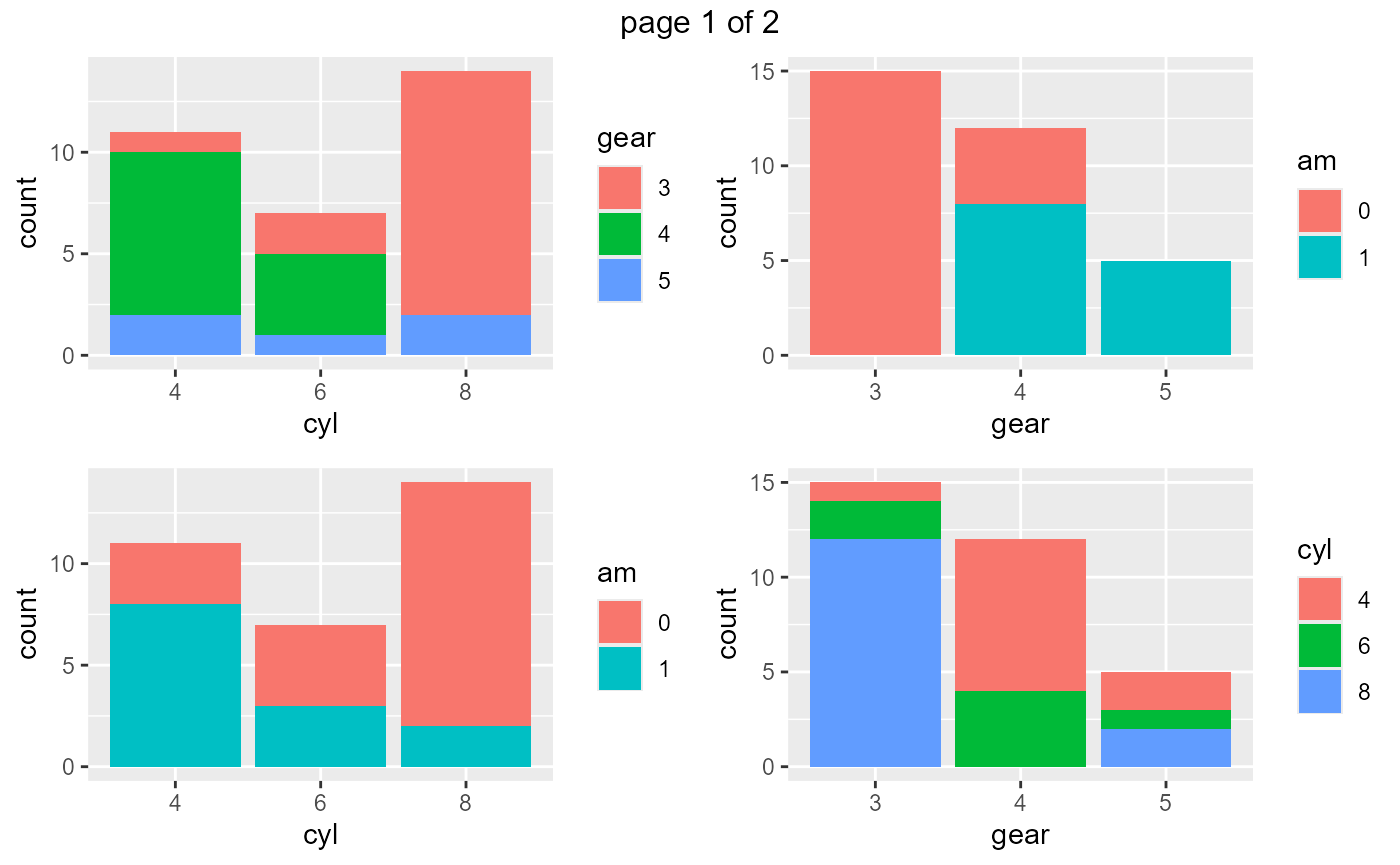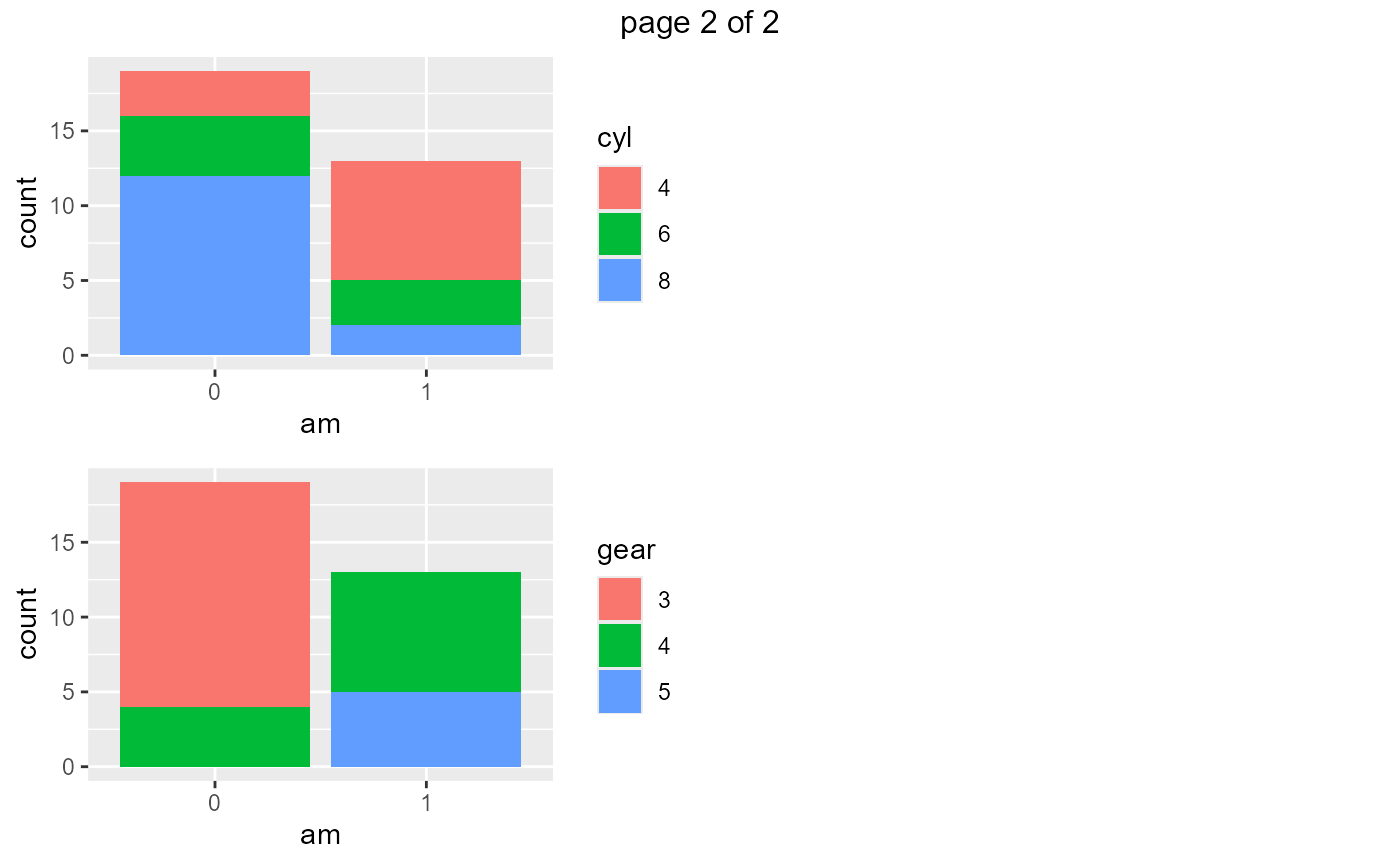Grouped Bar Plot

ds_plot_bar_grouped(mtcarz, cyl, gear, am)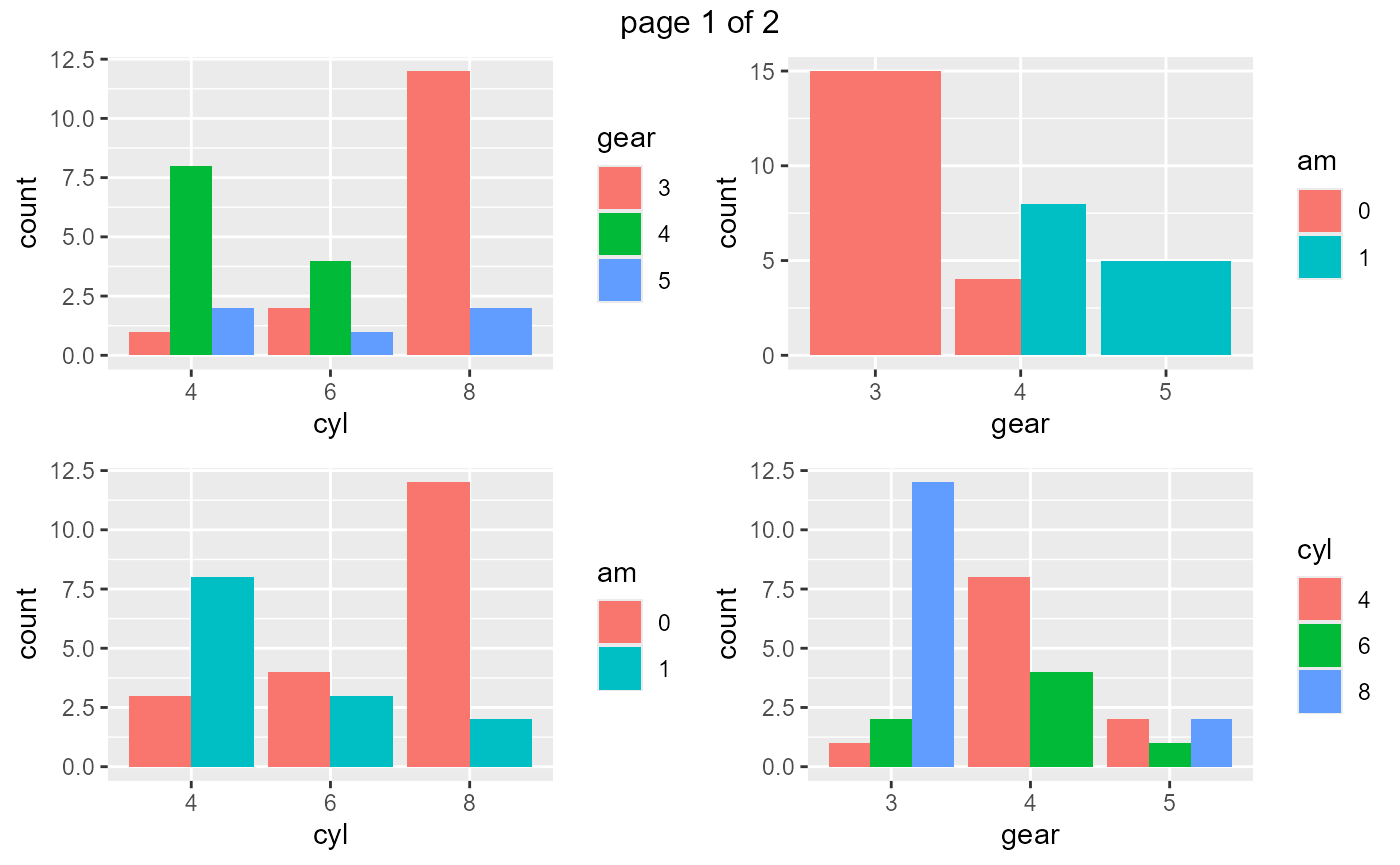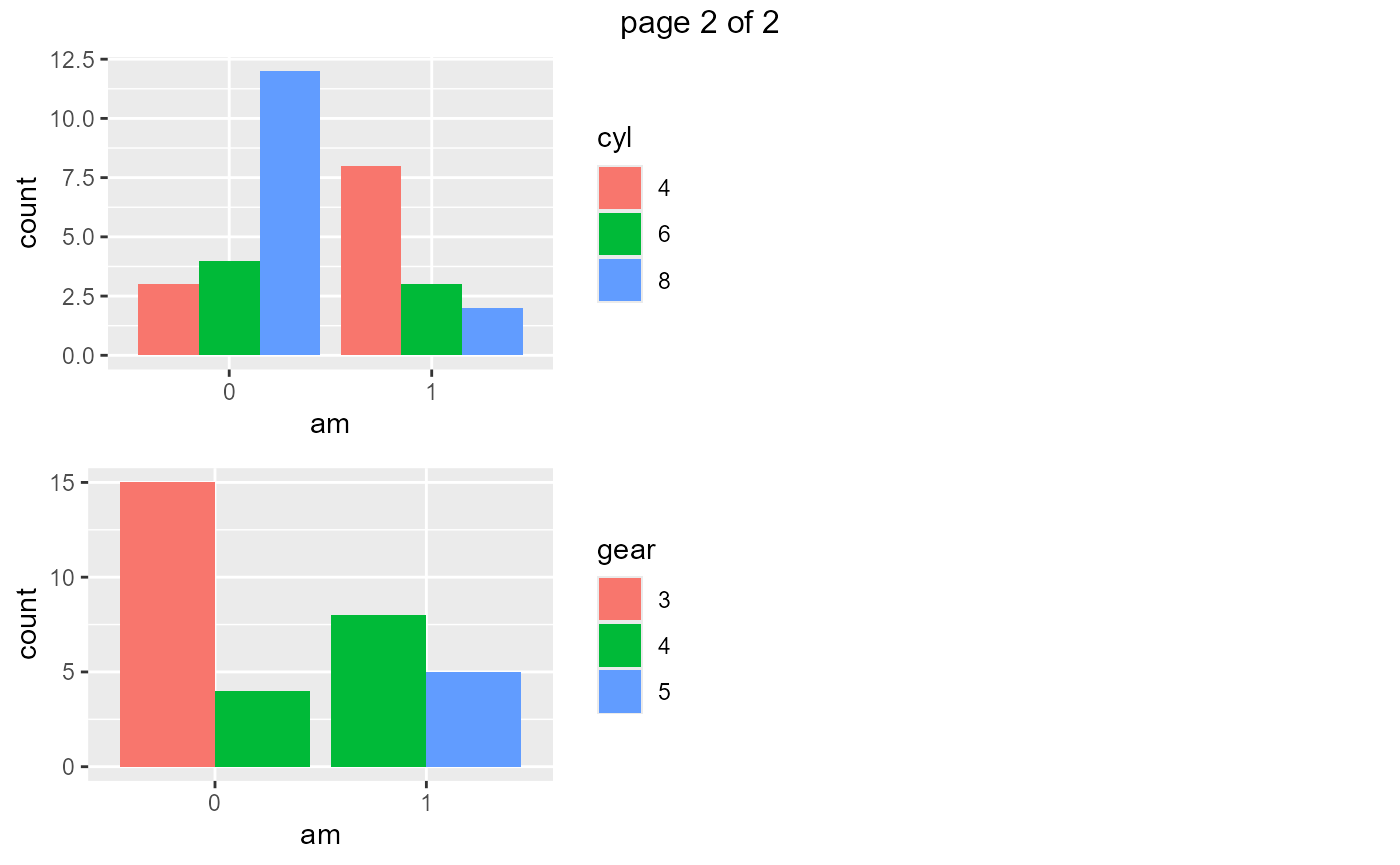Grouped Box Plots

ds_plot_box_group(mtcarz, cyl, gear, mpg, disp)